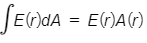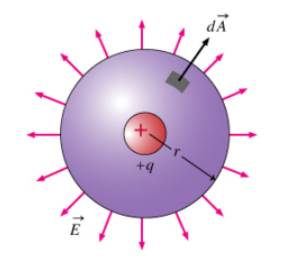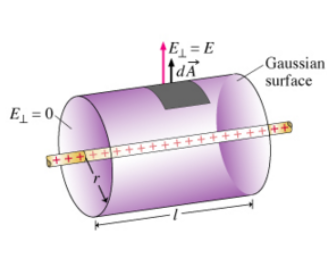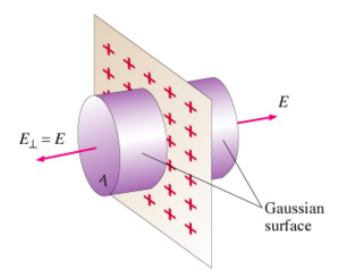# Problem: Consider a point charge g in three-dimensional space. Symmetry requires the electric field to point directly away from the charge in all directions. To find E(r), the magnitude of the field at distance r from the charge, the logical Gaussian surface is a sphere centered at the charge. The electric field is normal to the surface, so the dot product of the electric field and an infinitesimal surface element involves element involves cos(0) = 1. The flux integral is therefore reduced to , where E(r) is the magnitude of the electric field on the Gaussian surface, and A(r) is the area of the surface. Part ADetermine the magnitude E(r) by apliying Gauss's law. Part BBy symmetry, the electric field must point radially outward from the wire at each point; that is , the field lines lie in planes perpendicular to the wire. In solving for the magnitude of the radial electric field E(r) produced by a line charge with charge density λ, one should use a cylindrical Gaussian surfase whose axis is the line charge. The lenght of the cylindrical surface L should cancel out of the expression for E(r). Apply Gauss's law to this situation to find an expression for E(r). Part CIn solving for the magnitude of the electric field E(z) produced by a sheet charge with charge density δ, use the planar symmetry since the charge distribution doesn't change if you slide it in any direction of xy plane parallel to the sheet. Therefore at each point, the electric field is perpendicular to the sheet and must have the same magnitude at any given distance on either side of the sheet. To take advantage of these symmetry properties, use a Gaussian surface in the shape of a cylinder with its axis perpendicular to the sheet of charge, with ends of area A which wil cancel out the expression for E(z) in the end. The result of applying Gauss's law to this situation then gives an expression for E(z) for both z&gt;0 and z&lt;0.

###### FREE Expert Solution

From Gauss's law:

$\overline{){\mathbf{\oint }}{\mathbf{E}}{\mathbf{·}}{\mathbf{d}}{\mathbf{A}}{\mathbf{=}}{\mathbf{E}}{\mathbf{A}}{\mathbf{=}}\frac{\mathbf{q}}{{\mathbf{\epsilon }}_{\mathbf{0}}}}$

Part A

A = 4πr2

So we have:

$\begin{array}{rcl}\mathbf{E}\mathbf{\left(}\mathbf{4}\mathbf{\pi }{\mathbf{r}}^{\mathbf{2}}\mathbf{\right)}& \mathbf{=}& \frac{\mathbf{q}}{{\mathbf{\epsilon }}_{\mathbf{0}}}\\ \mathbf{E}\mathbf{\left(}\mathbf{r}\mathbf{\right)}& \mathbf{=}& \frac{\mathbf{q}}{\mathbf{4}\mathbf{\pi }{\mathbf{\epsilon }}_{\mathbf{0}}{\mathbf{r}}^{\mathbf{2}}}\end{array}$

87% (244 ratings)###### Problem Details

Consider a point charge g in three-dimensional space. Symmetry requires the electric field to point directly away from the charge in all directions. To find E(r), the magnitude of the field at distance r from the charge, the logical Gaussian surface is a sphere centered at the charge. The electric field is normal to the surface, so the dot product of the electric field and an infinitesimal surface element involves element involves cos(0) = 1. The flux integral is therefore reduced to, where E(r) is the magnitude of the electric field on the Gaussian surface, and A(r) is the area of the surface.

Part A

Determine the magnitude E(r) by apliying Gauss's law.Part B

By symmetry, the electric field must point radially outward from the wire at each point; that is , the field lines lie in planes perpendicular to the wire. In solving for the magnitude of the radial electric field E(r) produced by a line charge with charge density λ, one should use a cylindrical Gaussian surfase whose axis is the line charge. The lenght of the cylindrical surface L should cancel out of the expression for E(r). Apply Gauss's law to this situation to find an expression for E(r).Part C

In solving for the magnitude of the electric field E(z) produced by a sheet charge with charge density δ, use the planar symmetry since the charge distribution doesn't change if you slide it in any direction of xy plane parallel to the sheet. Therefore at each point, the electric field is perpendicular to the sheet and must have the same magnitude at any given distance on either side of the sheet. To take advantage of these symmetry properties, use a Gaussian surface in the shape of a cylinder with its axis perpendicular to the sheet of charge, with ends of area A which wil cancel out the expression for E(z) in the end. The result of applying Gauss's law to this situation then gives an expression for E(z) for both z>0 and z<0.